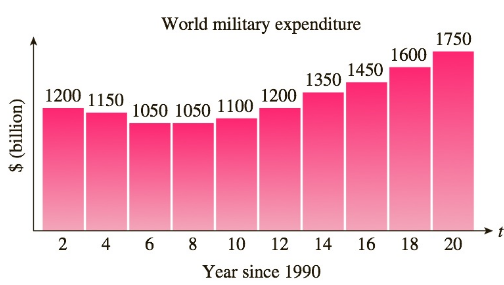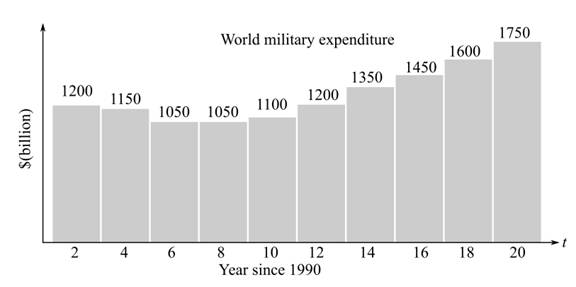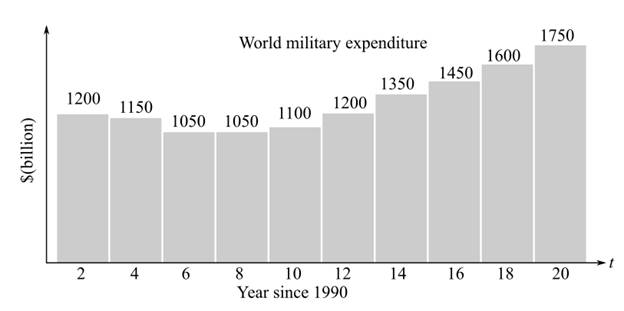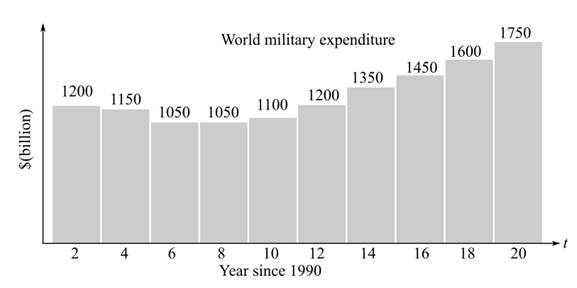Chapter 2.1, Problem 25EFinite Mathematics and Applied Cal...

7th Edition
Stefan Waner + 1 other
ISBN: 9781337274203

Solutions

Chapter
SectionFinite Mathematics and Applied Cal...

7th Edition
Stefan Waner + 1 other
ISBN: 9781337274203
Textbook Problem

World Military Expenditure The following chart shows total military and arms trade expenditure from 1992–2010 ( t = 0 represents 1990).2Source: www.globalissues.org/Geopolitics/ArmsTrade/Spending.asp.a. If you want to model the expenditure figures with a function of the form f ( t ) = a t 2 + b t + c , would you expect the coefficient a to be positive or negative? Why? [HINT: See “Features of a Parabola” in this section.]b. Which of the following models best approximates the data given? (Try to answer this without actually computing values.)A. f ( t ) = 4 t 2 − 56 t − 1 , 300 B. f ( t ) = − 4 t 2 − 56 t + 1 , 300 C. f ( t ) = 4 t 2 − 56 t + 1 , 300 D. f ( t ) = − 4 t 2 − 56 t − 1 , 300 c. What is the nearest year that would correspond to the vertex of the graph of the correct model from part (b)? What is the danger of extrapolating the data in either direction?

(a)

To determine

The nature of a from the following graph of expenditure as a function of time, of the form f(t)=at2+bt+c and graph is shown below:Explanation

Given Information:

The provided equation,

f(t)=at2+bt+c

The provided graph,

Consider the given graph,

(b)

To determine

The best quadratic model that can best represent the following data of expenditure as a function of time, of the form f(t)=at2+bt+c and graph is shown below,The provided options are:

(a). f(t)=4t256t1300

(b). f(t)=4t256t+1300

(c). f(t)=4t256t+1300

(d). f(t)=4t256t1300

(c)

To determine

The nearest year that would correspond to the vertex of the graph of the correct model. Also determine the danger of extrapolating the data shown below in either direction. The model for data of the form f(t)=at2+bt+c.Still sussing out bartleby?

Check out a sample textbook solution.

See a sample solution

The Solution to Your Study Problems

Bartleby provides explanations to thousands of textbook problems written by our experts, many with advanced degrees!

Get Started